# Trigonometry

Relations Between Trigonometric Functions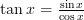$\tan x = \frac{\sin x}{\cos x}$ and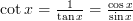$\cot x = \frac{1}{\tan x} = \frac{\cos x}{\sin x}$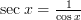$\sec x = \frac{1}{\cos x}$ and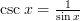$\csc x = \frac{1}{\sin x}$

Pythagorean Formula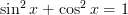$\sin ^2 x + \cos ^2 x = 1$

Negative Angles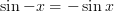$\sin -x = -\sin x$ and$\cos -x = \cos x$ and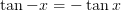$\tan -x = -\tan x$

Sum Formulas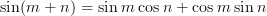$\sin (m+n) = \sin m \cos n + \cos m \sin n$ and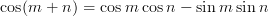$\cos (m+n) = \cos m \cos n - \sin m \sin n$